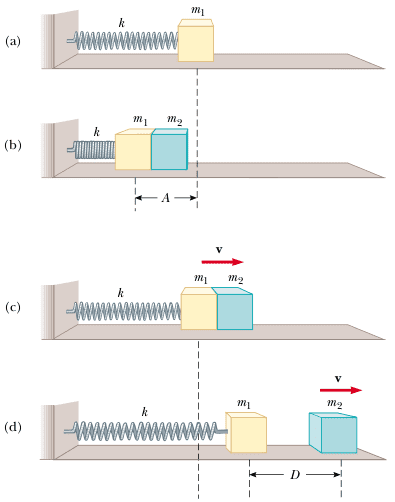# Spring Pushing Two Masses

• najd

## Homework Statement

Question statement:
An object of mass m1 = 9.00 kg is in equilibrium while connected to a light spring of constant s = 100 N/m that is fastened to a wall as shown in (figure a):A second object, m2 = 7.00 kg, is slowly pushed up against m1, compressing the spring by the amount A = 0.200 m (figure b). The system is then released, and both objects start moving to the right on the frictionless surface.

A- When m1 reaches the equilibrium point, m2 loses contact with m1 (figure c) and moves to the right with speed v. Determine the value of v.
B- How far apart are the objects when the spring is fully stretched for the first time (figure d)?

T= 0.5mv²
E_tot= 0.5sA²
v_max= Aω

## The Attempt at a Solution

Okay, so the amplitude for the coupled system is 0.2 metres. The angular frequency of them both is:
ω= √(s/(m1+m2)) = √(100/(16)) = 2.5/s

The velocity of the second mass would equal the maximum velocity reached by the two objects as they pass the equilibrium point, so:
v_max= Aω= 0.2*2.5 = 0.5m/s

So far so good, now the part which I'm unsure of:
To figure out the distance between them, first I calculated how far the spring will get stretched after m2 loses contact with m1.
1- I calculated T for m1:
T1= 0.5m1v² = 0.5*9*(0.5)^2= 1.125J

2- I used conservation of energy to calculate A:
E1_tot= T1 and so,
A= √(2T/s) = √(2*1.125/100) = 0.15m
[This turned out to be less than 0.2m, is that even logical? I mean, the amplitude decreased after m2 broke free. I imagine it should increase.. even though it looks smaller in the figure.]

3- I calculated the time needed for m1 to reach that position:
τ= 2∏/ω1 = 2∏√(m1/s) = 2∏√(9/100) = 1.88s for a whole cycle.
And so, the time for a quarter of a cycle would be:
1.88/4 = 0.47s

4- I calculated the distance traveled by m2:
d= vt = 0.5*0.47 = 0.235m

5- I subtracted the amplitude of m1 from the distance traveled by m2 to obtain D.
D= 0.235-0.15 = 0.085m.

Anything wrong with what I did?

[This turned out to be less than 0.2m, is that even logical? I mean, the amplitude decreased after m2 broke free. I imagine it should increase.. even though it looks smaller in the figure.]

I assume the amplitude decreased because the system lost part of its energy as m2 broke free, and so, it would make sense.. correct me if I'm wrong, please.